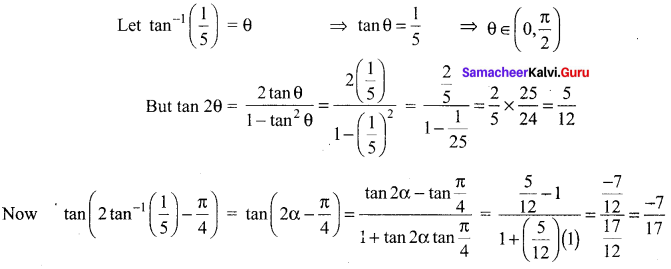## Tamilnadu Samacheer Kalvi 12th Maths Solutions Chapter 4 Inverse Trigonometric Functions Ex 4.4

Question 1.
Find the principal value of
(i) sec-1 ($$\frac{2}{\sqrt{3}}$$)
(ii) cot-1(√3)
(iii) cosec-1(-√2)
Solution:
(i) Let sec-1 ($$\frac{2}{\sqrt{3}}$$) = θ
⇒ sec θ = $$\frac{2}{\sqrt{3}}$$
⇒ cos θ = $$\frac{\sqrt{3}}{2}$$ = cos $$\frac{\pi}{6}$$
⇒ θ = $$\frac{\pi}{6}$$

(ii) Let cot-1(√3) = θ
⇒ cot θ = √3
⇒ tan θ = $$\frac{1}{\sqrt{3}}$$ = tan $$\frac{\pi}{6}$$
⇒ θ = $$\frac{\pi}{6}$$

(iii) Let cosec-1 (-√2) = θ
⇒ cosec θ = -√2
⇒ sin θ = $$-\frac{1}{\sqrt{2}}$$
⇒ θ = $$-\frac{\pi}{4}$$

Question 2.
Find the value of
(i) tan-1(√3) – sec-1(-2)
(ii) sin-1(-1) + cos-1 ($$\frac{1}{2}$$) + cot-1(2)
(iii) cot-1(1) + sin-1($$-\frac{\sqrt{3}}{2}$$) – sec-1(-√2)
Solution: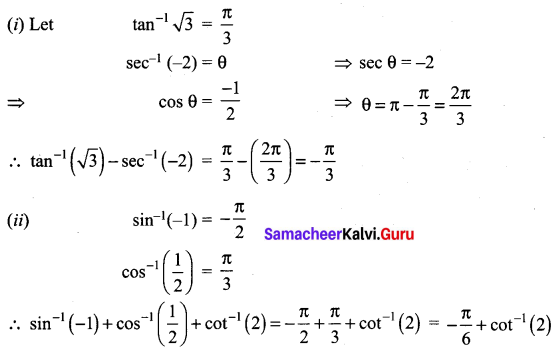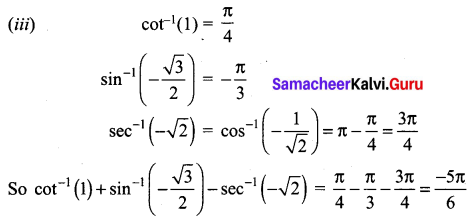### Samacheer Kalvi 12th Maths Solutions Chapter 4 Inverse Trigonometric Functions Ex 4.4 Additional Problems

Question 1.
Find the principal value of the following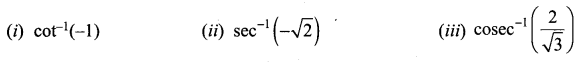Solution: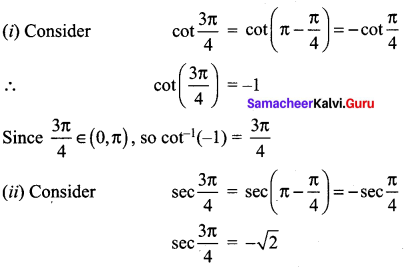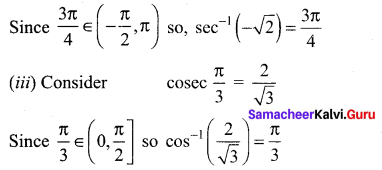Question 2.
Find the value of sec2(cot-1 3) + cosec2 (tan-1 2)
Solution: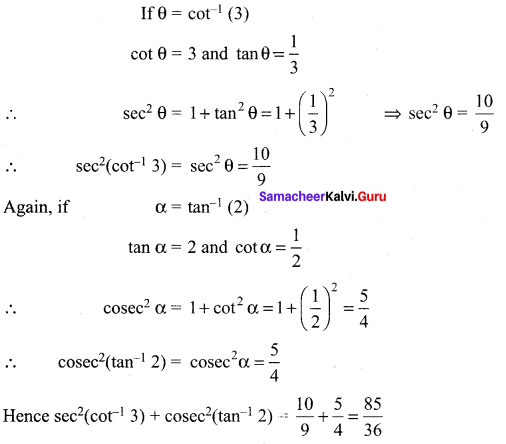Question 3.
Find the value of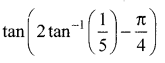Solution: